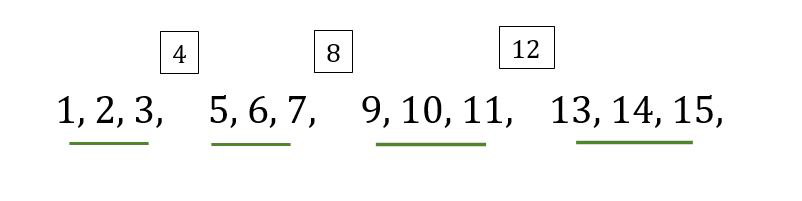# Find the Nth natural number which is not divisible by A

• Difficulty Level : Hard
• Last Updated : 21 Apr, 2021

Given two integers A and N, our task is to find the Nth natural number which is not divisible by A.

Examples:

Input: A = 4, N = 12
Output: 15
Explanation:
The series starting from 1 excluding the multiples of A would be 1, 2, 3, 5, 6, 7, 9, 10, 11, 13, 14, 15, 17 and the 12th term which is not divisible by 4 is 15.

Input: A = 3, N = 20
Output: 29
Explanation:
The series starting from 1 excluding the multiples of A would be 1, 2, 4, 5, 7, 8, 10, 11 and so on and the Nth number which is not divisible by 3 is 29.

Approach:

To solve the problem mentioned above we have to observe that there is a gap after every (A – 1) integer as the multiples of A are skipped. To find that the number N lies in which set we divide N by (A – 1), and store it in a variable lets say quotient. Now multiplying A with that variable and adding the remainder to it we will get the resultant answer.

```If A = 4, N = 7:
quotient = 7 / 3 = 2
remainder = 7 % 3 = 1

So the answer is
(A * quotient) + remainder
= 4 * 2 + 1 = 9```But if the remainder is 0 it means it is the last element in the set. Lets us understand it by an example.

```If A = 4, N = 6:
quotient = 6 / 3 = 2
remainder = 6 % 3 = 0

So the answer is
(A * quotient) - 1
= 4 * 2 - 1 = 7```

Below is the implementation of the above approach:

## C++

 `// C++ code to Find the Nth number which``// is not divisible by A from the series``#include``using` `namespace` `std;` `void` `findNum(``int` `n, ``int` `k)``{``    ` `    ``// Find the quotient and the remainder``    ``// when k is divided by n-1``    ``int` `q = k / (n - 1);``    ``int` `r = k % (n - 1);``    ``int` `a;` `    ``// If the remainder is not 0``    ``// multiply n by q and subtract 1``    ``// if remainder is 0 then``    ``// multiply n by q``    ``// and add the remainder``    ``if``(r != 0)``       ``a = (n * q) + r;``    ``else``       ``a = (n * q) - 1;` `    ``// Print the answer``    ``cout << a;``}` `// Driver code``int` `main()``{``    ``int` `A = 4, N = 6;``    ` `    ``findNum(A, N);``    ``return` `0;``}` `// This code is contributed by PratikBasu`

## Java

 `// Java code to Find the Nth number which``// is not divisible by A from the series``class` `GFG {``    ` `static` `void` `findNum(``int` `n, ``int` `k)``{` `    ``// Find the quotient and the remainder``    ``// when k is divided by n-1``    ``int` `q = k / (n - ``1``);``    ``int` `r = k % (n - ``1``);``    ``int` `a = ``0``;``    ` `    ``// If the remainder is not 0``    ``// multiply n by q and subtract 1``    ``// if remainder is 0 then``    ``// multiply n by q``    ``// and add the remainder``    ``if` `(r != ``0``)``        ``a = (n * q) + r;``    ``else``        ``a = (n * q) - ``1``;` `    ``// Print the answer``    ``System.out.println(a);``}` `// Driver code``public` `static` `void` `main(String[] args)``{``    ``int` `A = ``4``;``    ``int` `N = ``6``;` `    ``findNum(A, N);``}``}` `// This code is contributed by 29AjayKumar`

## Python3

 `# Python3 code to Find the Nth number which``# is not divisible by A from the series` `def` `findNum(n, k):``    ` `    ``# Find the quotient and the remainder``    ``# when k is divided by n-1``    ``q ``=` `k``/``/``(n``-``1``)``    ``r ``=` `k ``%` `(n``-``1``)``    ` `    ``# if the remainder is not 0``    ``# multiply n by q and subtract 1``    ` `    ``# if remainder is 0 then``    ``# multiply n by q``    ``# and add the remainder``    ``if``(r !``=` `0``):``        ``a ``=` `(n ``*` `q)``+``r``    ``else``:``        ``a ``=` `(n ``*` `q)``-``1``        ` `    ``# print the answer``    ``print``(a)` `# driver code``A ``=` `4``N ``=` `6``findNum(A, N)`

## C#

 `// C# code to find the Nth number which``// is not divisible by A from the series``using` `System;` `class` `GFG{``    ` `static` `void` `findNum(``int` `n, ``int` `k)``{` `    ``// Find the quotient and the remainder``    ``// when k is divided by n-1``    ``int` `q = k / (n - 1);``    ``int` `r = k % (n - 1);``    ``int` `a = 0;``    ` `    ``// If the remainder is not 0``    ``// multiply n by q and subtract 1``    ``// if remainder is 0 then``    ``// multiply n by q``    ``// and add the remainder``    ``if` `(r != 0)``        ``a = (n * q) + r;``    ``else``        ``a = (n * q) - 1;` `    ``// Print the answer``    ``Console.WriteLine(a);``}` `// Driver code``public` `static` `void` `Main(String[] args)``{``    ``int` `A = 4;``    ``int` `N = 6;` `    ``findNum(A, N);``}``}` `// This code is contributed by amal kumar choubey`

## Javascript

 ``

Output:

`7`

My Personal Notes arrow_drop_up